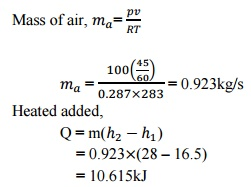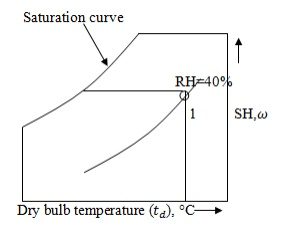Home | | Engineering Thermodynamics | Solved Problems: Gas Mixtures and Psychrometry

# Solved Problems: Gas Mixtures and Psychrometry

Mechanical - Engineering Thermodynamics - Gas Mixtures and Psychrometry

SOLVED PROBLEMS

1.     Atmospheric air at 1.0132bar has a DBT of 30°C and a WBT of 25°C. Compute

i.            The partial pressure of water vapour,

ii.           The specific humidity,

iii.           The dew point temperature,

iv.           The relative humidity,

v.           The degree of saturation,

vi.           The density of air in the mixture,

vii.          The density of vapour in the mixture and

viii.          The enthalpy of the mixture. Use thermodynamic table.2. Air at 20°C, 40% relative humidity is missed adiabatically with air at 40°C, 40% RH in the ratio of 1kg of former with 2kg of latter (on dry basis). Find the final condition (humidity and enthalpy) of air.3.An air conditioning system is to take in outdoor air at 283K and 30percent  relative humidity at a steady rate of 45 /min and to condition it to 298K and 60% relative humidity. The outdoor air is first heated to 295K in the heating section and then humidified by the injection of hot steam in the humidifying section. Assuming the entire process takes place at a pressure of 100kPa, determine (i) the rate of heat supply in the heating section and (ii) the mass flow rate of the steam required in the humidifying section.

Given data:

= 10°C

= 30%

= 25°C

=  60%

= 22°C

= 45 /min = 100kPa

Solution:

Step 1:

The dry of air i.e 10°C of dry bulb temperature and 30% relative humidity is marked on the psychrometric chart at point

1.     The horizontal line is drawn up to 22°C to obtain point

2.     The dry of air i.e. 25°C dry bulb temperature and 60% relative humidity is marked on the psychrometric chart at pointDraw an inclined line from point 1 to 2. Read enthalpies and specific humidity

values at point 1,2 and 3 from psychrometric chart.

At point 1, enthalpy                                 = 16.5kJ/kg

At point 2, enthalpy                                 = 28kJ/kgMass of air,=

= 0.923kg/s

=                                                               0.923  (28 –16.5)

=                                                               10.615kJ

Specific humidity,

=                                                               0.003kg/kg of dry air

=                                                               0.012kg/kg of dry air

= 0.012 –0.003 = 0.009kg/kg of dry air

We know that,

Specific humidity,Mass of steam                                          = 0.0083kg/s

4.     (i) What is the lowest temperature that air can attain in an evaporative cooler, if it enters at 1atm, 302K and 40% relative humidity? [Nov/Dec 2008]

Given data: p = 1bar T = 302K = 40%

Solution:

From steam table, corresponding to dry bulb temperature 29°C, saturation pressure is 4.004kPa

Relative humidity,= 1.601kPa

Lowest temperature that air attain in an evaporative cooler = 113.6°C which is corresponding to = 1.601kPa.

(ii) Consider a room that contains air at 1atm, 308K and 40% relative humidity. Using the psychrometric chart, determine: the specific humidity, the enthalpy, the wet bulb temperature, the dew point temperature and the specific volume of air.

Given data:

Pressure, p = 1atm

Relative humidity, = 40% Temperature, T = 308K = 35°C

Solution:

· Specific humidity,

From the point 1, draw a horizontal line with respect to temperature and relative humidity. At this point specific humidity is 0.0138kJ/kg. i.e. = 0.0138kJ/kg· Enthalpy, h and Wet bulb temperature,

From the point 1, draw a inclined line along the constant wet bulb temperature line till it cuts enthalpy line. At this point, enthalpy is 52.5kJ/kg and wet bulb temperature is 23.9°C. i.e. h = 52.5kJ/kg and

= 23.9°CMark point 1 on the psychrometric chart by given dry bulb temperature (35°C) and relative humidity 40%.

From point 1, draw a horizontal line to the left till it cuts saturation curve.

At that point, temperature is 20°C and specific volume is 0.89                   . i.e.

= 20°C and v = 0.89                           .

5.     30 /min of moist air at 15°C DBT and 13°C WBT are mixed with 12 /min of moist air at 25°C DBT and 18°C WBT. Determine DBT and WBT of the mixture assuming barometric pressure is one atmosphere.

Given data:

First steam of air,

Dry bulb temperature,                         = 15°C

Wet bulb temperature,                         = 13°C

Flow rate,                                            = 30     /min

Second steam of air,

Dry bulb temperature,                         = 25°C

Wet bulb temperature,                         = 18°C

Flow rate,                                            = 12     /min

Solution:

Step 1:

The first steam of air 15°C DBT and 13°C WBT is marked on the psychrometric chart at point 1.

Step 2:

The second stream of air 25°C DBT and 18°C WBT is marked on the psychrometric chart at point 2.

Join the points 1 and 2 from psychrometric chart. Step 3:We know that,

Specific humidity of first steam of air, = 0.007kg/kg of dry air

Specific humidity of second steam of air,= 0.00943kg/kg of dry air Specific humidity after mixing,

= 0.00943kg/kg of dry air

Step 5:

Draw a horizontal line from          = 0.00943 till it cuts 1 –2 line.

Name the point 3.

From psychrometric chart, at point 3

Dry bulb temperature,                  = 24.02°C

Wet bulb temperature,                  = 18.2°C

Study Material, Lecturing Notes, Assignment, Reference, Wiki description explanation, brief detail
Mechanical : Engineering Thermodynamics : Gas Mixtures and Psychrometry : Solved Problems: Gas Mixtures and Psychrometry |

Related Topics